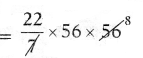# AP Board 7th Class Maths Solutions Chapter 11 Area of Plane Figures Ex 11.3

SCERT AP 7th Class Maths Solutions Pdf Chapter 11 Area of Plane Figures Ex 11.3 Textbook Exercise Questions and Answers.

## AP State Syllabus 7th Class Maths Solutions 11th Lesson Area of Plane Figures Ex 11.3

Question 1.
The diameter of the round table upper surface in the Science lab is 70 cm. Find the area.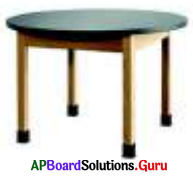Given diameter of table d = 70 cm
Radius of table r = $$\frac{\mathrm{d}}{2}$$ = $$\frac{70}{2}$$ = 35 cm
Area of the table = πr2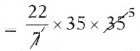∴ Area of the round table = 3,850 sq.cm.Question 2.
Radius of the circular shaped wall painting is 14 cm. Calculate the area of wall painting.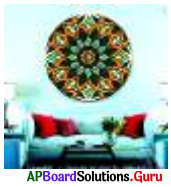Given radius of the wall painting
r = 14 cm
Area of the circle = πr2
= $$\frac{22}{7}$$ × 142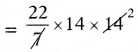= 616
∴ Area of the wall painting = 616 sq.cm

Question 3.
If the area of circular shaped dart board is 1386 sq.cm. Find its radius and diameter.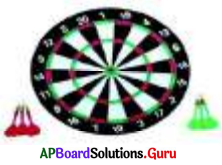Given the area of circular shaped dart board = 1386 sq.cm
Area of the circle = πr2 = 1386
⇒ $$\frac{22}{7}$$ × r2 = 1386
⇒ 22 × r2 = 1386 × 7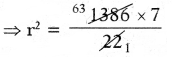⇒ r2 = 441 = 212
⇒ r2 = 212
∴ Radius of dart board r = 21 cm
Diameter of dart board d 2r
= 2 × 21
= 42 cmQuestion 4.
Circumference of the circular shaped clock is 44 cm. Find the radius and surface area of the clock.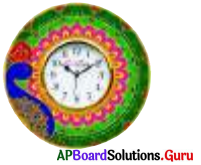Given the circumference of clock = 44 cm
⇒ 2πr = 44 cm
⇒ 2 × $$\frac{22}{7}$$ × r = 44
⇒ 44 × r = 44 × 7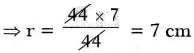∴ Radius of the clock r = 7 cm
Surface area of the clock = πr2
= $$\frac{22}{7}$$ × 72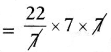∴ Surface area of the clock = 154 sq.cmQuestion 5.
The circumference of a circular-shaped lawn in a park is 352 m. Find the area of the circular-shaped lawn. If the cost of grass per sq. m is ₹ 30, then find the total cost of laying grass on the lawn.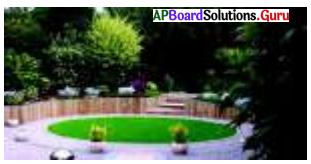⇒ 2 × $$\frac{22}{7}$$ × r = 352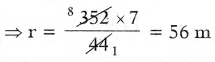= $$\frac{22}{7}$$ × 562